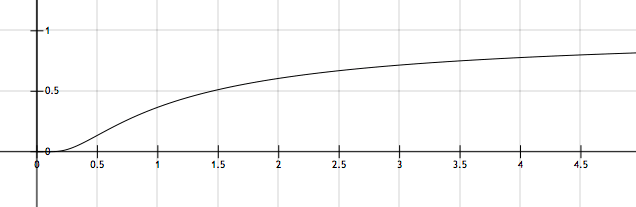# They call it chemical kinetics?

$\large k = A e^{-\frac{E_a}{RT}}$

The Arrhenius equation above tell us how the rate constant $k$ for a particular reaction depends on its activation energy $E_a$ and temperature $T:$The figure above shows a typical plot of $k$ versus $T$. If the corresponding value of $k$ for the point of inflection for the curve above is given by $k' = A e^X$ for some constant $X,$ then find $|X|.$

Assumptions and Clarifications:

1. The pre-exponential factor or $A$ for the given reaction is essentially a constant.
2. $R=8.314 \text{ J/(K mol)}$ is the gas constant.
×